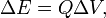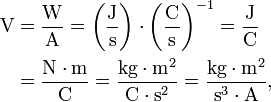# Volt

The volt (symbol V) is the SI unit of electrical potential and of electromotive force. The volt is named for an early pioneer of the science of electricity, Alessandro Volta (1745-1827).

One volt is the potential across which a current of one ampere will dissipate one watt of power. Namely, from the equation,$P = i\, V,$

where i is electric current (in ampere) and dissipated power is P (in watt = J/s), follows that the unit of V is watt/ampere (W/A).

The equation$\Delta E = Q \Delta V, \,$

where ΔE is the work to move a charge Q over a difference in electric potential field of ΔV, shows that V is the potential across which one coulomb of charge will gain or lose one joule of energy. Hence another unit of V is joule/coulomb (J/C).

The volt is a derived unit in the SI, equal to 1 W/A. In terms of some other SI units:\begin{align} \textrm{V} &= \frac{\textrm{W}}{\textrm{A}} = \left( \frac{\textrm{J}}{\textrm{s}}\right) \cdot \left(\frac{\textrm{C}}{\textrm{s}}\right)^{-1} = \frac{\textrm{J}}{\textrm{C}} \\ & = \frac{\textrm{N}\cdot\textrm{m}}{\textrm{C}} = \frac{\textrm{kg}\cdot\textrm{m}^2 }{\textrm{ C}\cdot\textrm{s}^{2}} = \frac{ \textrm{kg}\cdot\textrm{m}^2 }{\textrm{s}^{3} \cdot \textrm{A}}, \end{align}.

where the righmost side is in SI base units.

## Earlier definitions

The first definition of the volt was based on the cgs electromagnetic system of units. The unit of potential in the cgs electromagnetic system was too small for practical use by engineers, and the volt was defined as 108 cgs units of electrical potential.

In 1893, a new definition of the volt was made in electrochemical terms; a Clark cell (which uses mercury and zinc) operating at a temperature of 15oC was defined to produce 1.434 V of potential. The number 1.434 was chosen to make the new volt as close to the cgs-derived definition as could be determined at the time.

Future definitions of the volt made it a derived unit: at first, the volt was defined as the potential which would drive a current of one ampere over a resistance of one ohm, and later, the current definition, with minor redefinitions of the ampere slightly changing the size of the volt.

## Practical uses

Most small commercially available dry cells are either 1.5V cells, or batteries made up of several 1.5 volt cells in series, producing 3, 6, 9 or 12 volts. Automobile batteries (with some exceptions) are rated to produce 12 volts, though they will yield more than that when freshly charged. In the United States, residential electric service is usually 110 to 120 V(AC), with occasional 220V or 440V circuits for appliances. In much of Europe, the standard residential electrical service is 240V AC.

## Sources

• volt. Sizes.com (2003-10-29). Retrieved on 2007-04-16.
• abvolt. Sizes.com (2002-10-01). Retrieved on 2007-04-16.Some content on this page may previously have appeared on Citizendium.